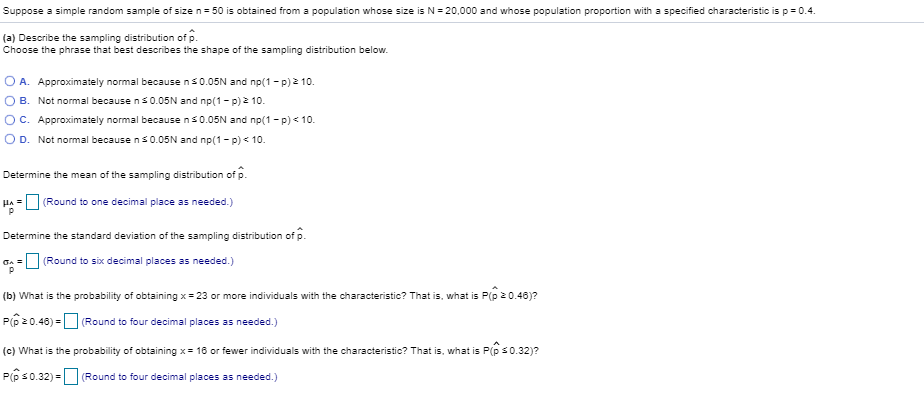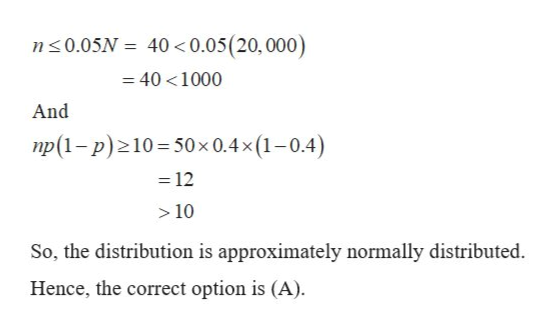Suppose a simple random sample of size n 50 is obtained from a population whose size is N20,000 and whose population proportion with a specified characteristic is p 0.4(a) Describe the sampling distribution of pChoose the phrase that best describes the shape of the sampling distribution belowOA. Approximately normal because ns0.05N and np(1 - p) 2 10O B. Not normal because ns 0.05N and np(1 - p)2 10.O C. Approximately normal because ns0.05N and np(1 - p)< 10O D. Not normal because ns0.05N and np(1- p) 10Determine the mean of the sampling distribution of p.(Round to one decimal place as needed.)HADetermine the standard deviation of the sampling distribution of p.(Round to six decimal places as needed.)(b) What is the probability of obtaining x 23 or more individuals with the characteristic? That is, what is P(p 0.46)?P20.46)=(Round to four decimal places as needed.)(c) What is the probability of obtaining x 16 or fewer individualss with the characteristic? That is, what is P(p s0.32)?PG S0.32)=(Round to four decimal places as needed.)

Questionhelp_outlineImage TranscriptioncloseSuppose a simple random sample of size n 50 is obtained from a population whose size is N20,000 and whose population proportion with a specified characteristic is p 0.4 (a) Describe the sampling distribution of p Choose the phrase that best describes the shape of the sampling distribution below OA. Approximately normal because ns0.05N and np(1 - p) 2 10 O B. Not normal because ns 0.05N and np(1 - p)2 10. O C. Approximately normal because ns0.05N and np(1 - p)< 10 O D. Not normal because ns0.05N and np(1- p) 10 Determine the mean of the sampling distribution of p. (Round to one decimal place as needed.) HA Determine the standard deviation of the sampling distribution of p. (Round to six decimal places as needed.) (b) What is the probability of obtaining x 23 or more individuals with the characteristic? That is, what is P(p 0.46)? P20.46)= (Round to four decimal places as needed.) (c) What is the probability of obtaining x 16 or fewer individualss with the characteristic? That is, what is P(p s0.32)? PG S0.32)= (Round to four decimal places as needed.) fullscreen
Step 1

The provided information are:

Population size, (N) = 20,000

Sample size (n) = 50

Population proportion (p) = 0.4

(a)

Sampling distribution of is said to be approximately normal distributed, if following conditions are satisfied:

Step 2

Now substitute the provided values in above conditions as:help_outlineImage Transcriptionclosen0.05N 40 0.05(20, 000) 40 1000 And np(1-p) 10 50x 0.4x (1-0.4) 12 10 So, the distribution is approximately normally distributed. Hence, the correct option is (A). fullscreen
Step 3

Mean of the sampling distribution of p...

Want to see the full answer?

See Solution

Want to see this answer and more?

Our solutions are written by experts, many with advanced degrees, and available 24/7

See Solution
Tagged in

Advanced Topics in Statistics